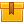# Principles of Reverse Double Dial Alignment (A8305)

## Prerequisites

This lesson is designed for participants familiar with precision measuring instruments, specifically dial indicators. A basic understanding of the principles and practices of predictive maintenance is also required.

## Description

This lesson describes the principles of the reverse double dial alignment method used to measure and correct misalignment. The lesson also explains the data needed to determine offset and angularity misalignment values by using a graph. Formulas used to calculate misalignment corrections and factors that may affect alignment are also presented.

## Objectives

Identify three types of misalignment found in most rotating equipment. Distinguish vertical plane from horizontal plane. Identify the parts of the alignment rig and explain how they work. Convert sweep reading to offset values. Plot indicator offset values on a graph to find angular and offset misalignment values. Read specifications correctly. Perform calculations to determine how far and in which direction to move the machine.

## ContentThis lesson describes the principles of the reverse double dial alignment method used to measure and correct misalignment. The lesson also explains the data needed to determine offset and angularity misalignment values by using a graph. Formulas used to calculate misalignment corrections and factors that may affect alignment are also presented.

•Principles of Reverse Double Dial Alignment (A8305)

0
0 Reviews